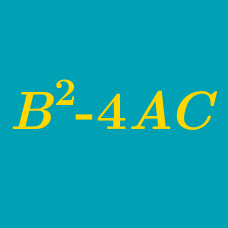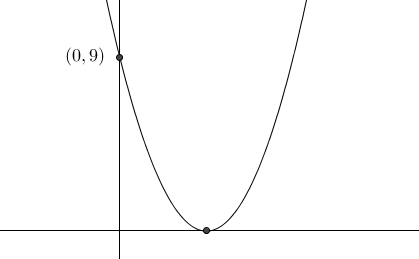Algebra

# Quadratic Discriminant: Level 2 Challenges

Find the positive value of $p$ such that the quadratic equation $px^2 - 12x + 4 = 0$ has only one solution.

$f(x)=ax^2+bx+c$

If $b>10, 0, how many times does the graph $y=f(x)$ cross the x-axis?If the curve $y=x^2+bx+c$ touches the $x$-axis at some point and intersects the y-axis at $(0,9)$, find the absolute value of $b$.

The difference of the roots of the quadratic equation $x^2 + bx + c = 0$ is -2. Find the discriminant of the given equation.

Find the positive value of $k$ such that the quadratic equation $4x^2+kx+9=0$ has identical roots.

×